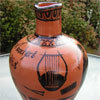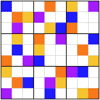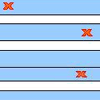Search by Topic

Resources tagged with Fractions similar to Modular Fractions:

Filter by: Content type:
Age range:
Challenge level:

There are 20 results

Broad Topics > Fractions, Decimals, Percentages, Ratio and Proportion > FractionsModular Fractions

Age 16 to 18 Challenge Level:

We only need 7 numbers for modulus (or clock) arithmetic mod 7 including working with fractions. Explore how to divide numbers and write fractions in modulus arithemtic.Look Before You Leap

Age 16 to 18 Challenge Level:

Relate these algebraic expressions to geometrical diagrams.Can it Be

Age 16 to 18 Challenge Level:Stretching Fractions: A Discussion

Age 14 to 18

This article extends and investigates the ideas in the problem "Stretching Fractions".What a Joke

Age 14 to 16 Challenge Level:

Each letter represents a different positive digit AHHAAH / JOKE = HA What are the values of each of the letters?More Twisting and Turning

Age 11 to 16 Challenge Level:

It would be nice to have a strategy for disentangling any tangled ropes...All Tangled Up

Age 14 to 18 Challenge Level:

Can you tangle yourself up and reach any fraction?Six Notes All Nice Ratios

Age 14 to 16 Challenge Level:

The Pythagoreans noticed that nice simple ratios of string length made nice sounds together.Harmonic Triangle

Age 14 to 16 Challenge Level:

Can you see how to build a harmonic triangle? Can you work out the next two rows?Fracmax

Age 14 to 16 Challenge Level:

Find the maximum value of 1/p + 1/q + 1/r where this sum is less than 1 and p, q, and r are positive integers.Rationals Between...

Age 14 to 16 Challenge Level:

What fractions can you find between the square roots of 65 and 67?Tangles

Age 11 to 16

A personal investigation of Conway's Rational Tangles. What were the interesting questions that needed to be asked, and where did they lead?Pythagoras’ Comma

Age 14 to 16 Challenge Level:

Using an understanding that 1:2 and 2:3 were good ratios, start with a length and keep reducing it to 2/3 of itself. Each time that took the length under 1/2 they doubled it to get back within range.Ratio Sudoku 3

Age 11 to 16 Challenge Level:

A Sudoku with clues as ratios or fractions.F'arc'tion

Age 14 to 16 Short Challenge Level:

At the corner of the cube circular arcs are drawn and the area enclosed shaded. What fraction of the surface area of the cube is shaded? Try working out the answer without recourse to pencil and. . . .Counting Fish

Age 14 to 16 Challenge Level:

I need a figure for the fish population in a lake. How does it help to catch and mark 40 fish?Stretching Fractions

Age 14 to 16 Challenge Level:

Imagine a strip with a mark somewhere along it. Fold it in the middle so that the bottom reaches back to the top. Stetch it out to match the original length. Now where's the mark?Equal Temperament

Age 14 to 16 Challenge Level:

The scale on a piano does something clever : the ratio (interval) between any adjacent points on the scale is equal. If you play any note, twelve points higher will be exactly an octave on.The Genes of Gilgamesh

Age 14 to 16 Challenge Level:

Can you work out the parentage of the ancient hero Gilgamesh?Circuit Training

Age 14 to 16 Challenge Level:

Mike and Monisha meet at the race track, which is 400m round. Just to make a point, Mike runs anticlockwise whilst Monisha runs clockwise. Where will they meet on their way around and will they ever. . . .